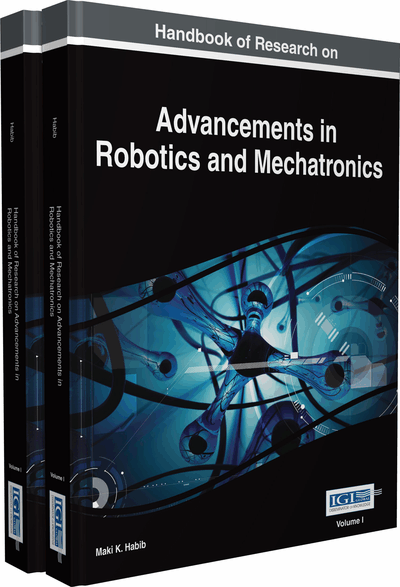# Dynamic Modeling and Control Techniques for a Quadrotor

Heba Elkholy (American University in Cairo, Egypt) and Maki K. Habib (The American University in Cairo, Egypt)
DOI: 10.4018/978-1-4666-7387-8.ch014
Available
\$29.50
No Current Special Offers

## Abstract

This chapter presents the detailed dynamic model of a Vertical Take-Off and Landing (VTOL) type Unmanned Aerial Vehicle (UAV) known as the quadrotor. The mathematical model is derived based on Newton Euler formalism. This is followed by the development of a simulation environment on which the developed model is verified. Four control algorithms are developed to control the quadrotor's degrees of freedom: a linear PID controller, Gain Scheduling-based PID controller, nonlinear Sliding Mode, and Backstepping controllers. The performances of these controllers are compared through the developed simulation environment in terms of their dynamic performance, stability, and the effect of possible disturbances.
Chapter Preview
Top

## State Of The Art

Controlling the degrees of freedom of the quadrotor can be done through various control algorithms which vary from the classical linear Proportional-Integral-Derivative (PID) or Proportional-Derivative (PD) controller to more complex nonlinear schemes such as backstepping or sliding mode controllers. Starting with the linear control algorithms; Bouabdallah et al. applied a PID and LQ controllers on an indoor micro quadrotor. The performance of the two controllers was comparable in stabilizing the attitude of the quadrotor around its hover position and under the effect of little disturbances [Bouabdallah et al. (2004)]. Li and Li used the classical PID to control the position and orientation of a quadrotor and it was able to stabilize in a low speed wind environment [Li & Li (2011)]. Simulation based results showed that Yang et al. were able to control the attitude and heading of a quadrotor using a self-tuning PID controller based on adaptive pole placement [Yang et al. (2013)]. Raffo et al. used an H controller to stabilize the rotational angles and a Model Predictive Controller (MPC) to track the desired position of a quadrotor. The effect of wind and model uncertainties was added to the simulated model and it performed robustly with a zero steady-state error [Raffo et al. (2010)].

In order to employ a linear controller to control a nonlinear system like that of the quadrotor, the system’s nonlinearities can be modeled as a collection of simplified linear systems and for each system a separate controller can be designed, this is the concept of gain scheduling and it is commonly used in flight controllers. Gillula et al. divided the state space model of a STARMAC quadrotor to a set of simple hybrid modes and this approach enabled the quadrotor to carry out aerobatic maneuvers [Gillula et al. (2011)]. Ataka et al. used gain scheduling on a linearized model of the quadrotor around some equilibrium points and tested the controllability and observability of the resulting system [Ataka et al. (2013)]. Amoozgar et al. compared the performance of a conventional PID controller to that of a gain scheduled PID controller with its parameters tuned using a fuzzy logic based inference scheme. The gain scheduled PID controller outperformed the conventional PID controller when the system was tested under actuator fault conditions [Amoozgar et al. (2012)]. In load dropping applications, Sadeghzadeh et al. found that a gain scheduled PID controller was able to stabilize the system during the dropping operation [Sadeghzadeh et al. (2012)].

## Key Terms in this Chapter

Proportional Integral Derivative (PID) Controller: A linear controller that can be used to control any dynamic system given its model, it consists of a proportional, an integral and a derivative term.

Unmanned Aerial Vehicle (UAV): A flying vehicle that is flown without a pilot which can be controlled autonomously or remotely.

Backstepping Controller: A recursive nonlinear controller that can be used to control a dynamic system given its model.

Sliding Mode Controller (SMC): A nonlinear controller of a switching nature that can be used to control any dynamic system given its model.

Genetic Algorithm: An iterative optimization algorithm that works to minimize a given objective function by generating a random population and performing genetic operations to generate a new population.

Proportional Derivative (PD) Controller: A simplification of a PID controller, consisting only of a proportional and a derivative term.

Quadrotor: A class of UAV that flies with the aid of four separately powered rotors.

Gain Scheduling Control: An adaptive control approach that is used to control nonlinear systems by deploying a set of linear controllers for different linear operating regions.

## Complete Chapter List

Search this Book:
Reset